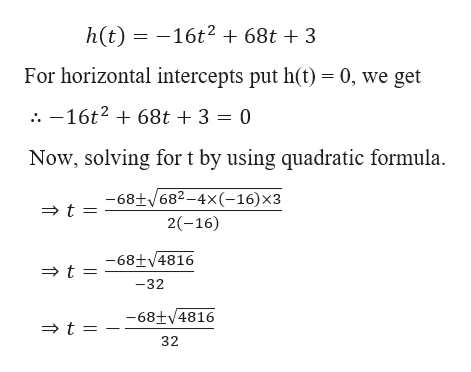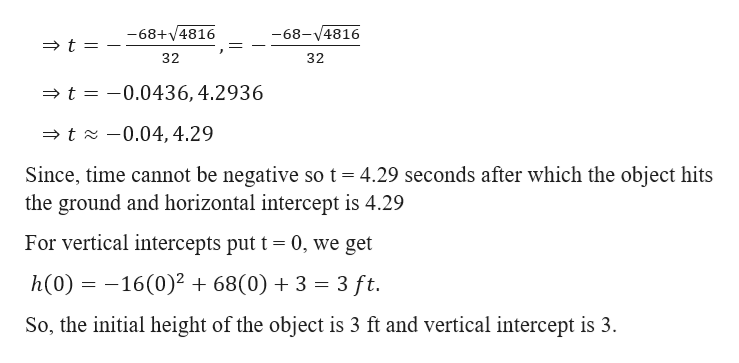# Find and interpret the horizontal and vertical intercepts for the following height equation.Round your answers to two decimal places when necessary.h=-16t2+68t+3 (h is in feet and t is in seconds)The initial height is Enter your answer; The initial height is _ feetfeet and the object hits the ground afterEnter your answer; the object hits the ground after _ secondsseconds.

Question
10 views

Find and interpret the horizontal and vertical intercepts for the following height equation.

h=-16t2+68t+3 (h is in feet and t is in seconds)

 The initial height is Enter your answer; The initial height is _ feet feet and the object hits the ground after Enter your answer; the object hits the ground after _ seconds seconds.

check_circle

Step 1

Given,help_outlineImage Transcriptionclose-16t2 +68t + 3 h(t) For horizontal intercepts put h(t) 0, we get . -16t2 68t +3 = 0 Now, solving for t by using quadratic formula -68+682-4x(-16)x3 t 2(-16) -68+ 4816 t -32 -68+4816 t 32 fullscreen
Step 2

Further calcul...help_outlineImage Transcriptionclose-68+v4816 -68-4816 t 32 32 t =-0.0436,4.2936 t-0.04, 4.29 Since, time cannot be negative so t 4.29 seconds after which the object hits the ground and horizontal intercept is 4.29 For vertical intercepts put t = 0, we get h(0) 16(0)2 68(0)3 3 ft. So, the initial height of the object is 3 ft and vertical intercept is 3. fullscreen

### Want to see the full answer?

See Solution

#### Want to see this answer and more?

Solutions are written by subject experts who are available 24/7. Questions are typically answered within 1 hour.*

See Solution
*Response times may vary by subject and question.
Tagged in
MathAlgebra

### Equations and In-equations